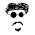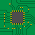## Adjustable Output Voltage Regulator using 7805

by realfinetime  |  in Voltage Regulator at  22:41

I had already published my blog on 5V Fixed-Output Voltage Regulator using 7805. 78xx series ICs can also be used to create adjustable voltage regulator. This blog will give you some tips to create adjustable voltage regulator using 7805. Circuit is done as shown in the following diagram.
7805 is capable of handling an input voltage from 7.5V to 25V. I gave an input voltage of 9V from an SMPS. A 0.33 micro Farad capacitor is connected across the input and a 0.1 micro Farad capacitor is connected across the output. R1 is fixed and R2 is variable. I kept R1 at 420 Ohms. Similarly a 0 - 200 Ohm variable resistor is used as R2. Output voltage is normally measured using a multimeter. Circuit can be summarised as given below.

7805                -  Voltage Regulator
Input Voltage  -  9V, 1A supply from an SMPS
R1                   -  420 Ohms
R2                   -  0 - 200 Ohm Variable
Capacitors       -  0.33 micro Farad at input side and 0.1 micro Farad at output side.

Output voltage varies with the R2 resistance value. Output voltage can be calculated using a simple formula.

Vo    -  Output Voltage
Vxx  -  Nominal output voltage ( Output to Common ) of fixed regulator. Vxx is 5V for 7805, 8V for 7808 and 12V for 7812.
Io      -  Output Current.

Procedure

Initially, keep the resistance R2 at minimum position ( 0 Ohm ). Then Vo = Vxx. That is, output voltage will be equal to nominal output voltage ( 5V for 7805 ). Now put R2 at maximum position ( 200 Ohms ). Then measure the output voltage using a multimeter. I got an output voltage of 8.14V. Calculation can be summarised as given below.

Vo    =    8.14V
Vxx  =    5.07V
R1    =    420 Ohms
R2    =    200 Ohms

Substituting these values in the above formula, we will get Io. Io will be same for a particular load, at different voltages. Using this value of  Io, Vxx and R1, we can calculate the value of  resistance R2 to be used for a desired value of Vo. Vo measured for various values of R2 using multimeter is given below.

Inference

From the table, it is clear that, Vo increase with increase in R2. So we will get a variable output voltage from 5.07V to 8.14V. An increase in the value of resistances above the values given in the table didn't give me an expected result.

1.Hello,
This is a great concept. Thank you for sharing this brilliant sharing. This post is very helpful and informative. Hope you post your next article soon.

2.78xx are FIXED voltage regulators, this could damage not only chip but also the application circuit. This is a very bad idea.

1.This is the exact circuit and formula published in TI's datasheet for the LM78xx series regulators. It is a safe and valid application. Thanks to www.learnerswings.com for walking us through a real world application.

3.LM317T is best for variable regulator. So you can drop to 1.25V output.

4.In this circuit, input 12 R1=? R2=? output=12V.
Using 12V 7ah Battery for dsl router.

5.Thank you ...

## IMPORTANT NOTICE

All the circuits, published in this blog is only after testing and getting proper results in my private lab. When you try these circuits, you should check the supply voltage, polarity of components, presence of childrens nearby and shorts in the circuits. This website will not be responsible for any harm happened to you or your components caused by your carelessness.